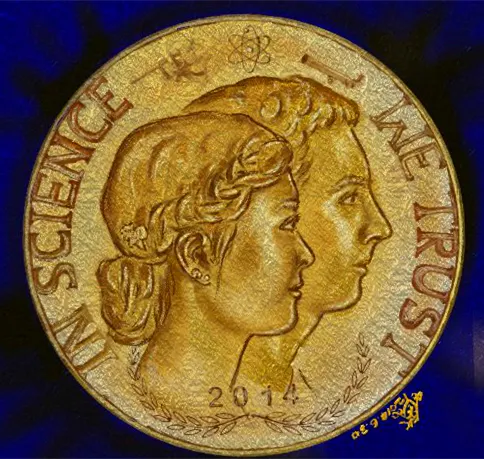# Mother and Child - DFRobot Bluno Beetle

A pair of Bluetooth connected flower brooches sends alert to mother when the child is out of proximity. When close by, both light up.

IntermediateFull instructions provided24 hours1,119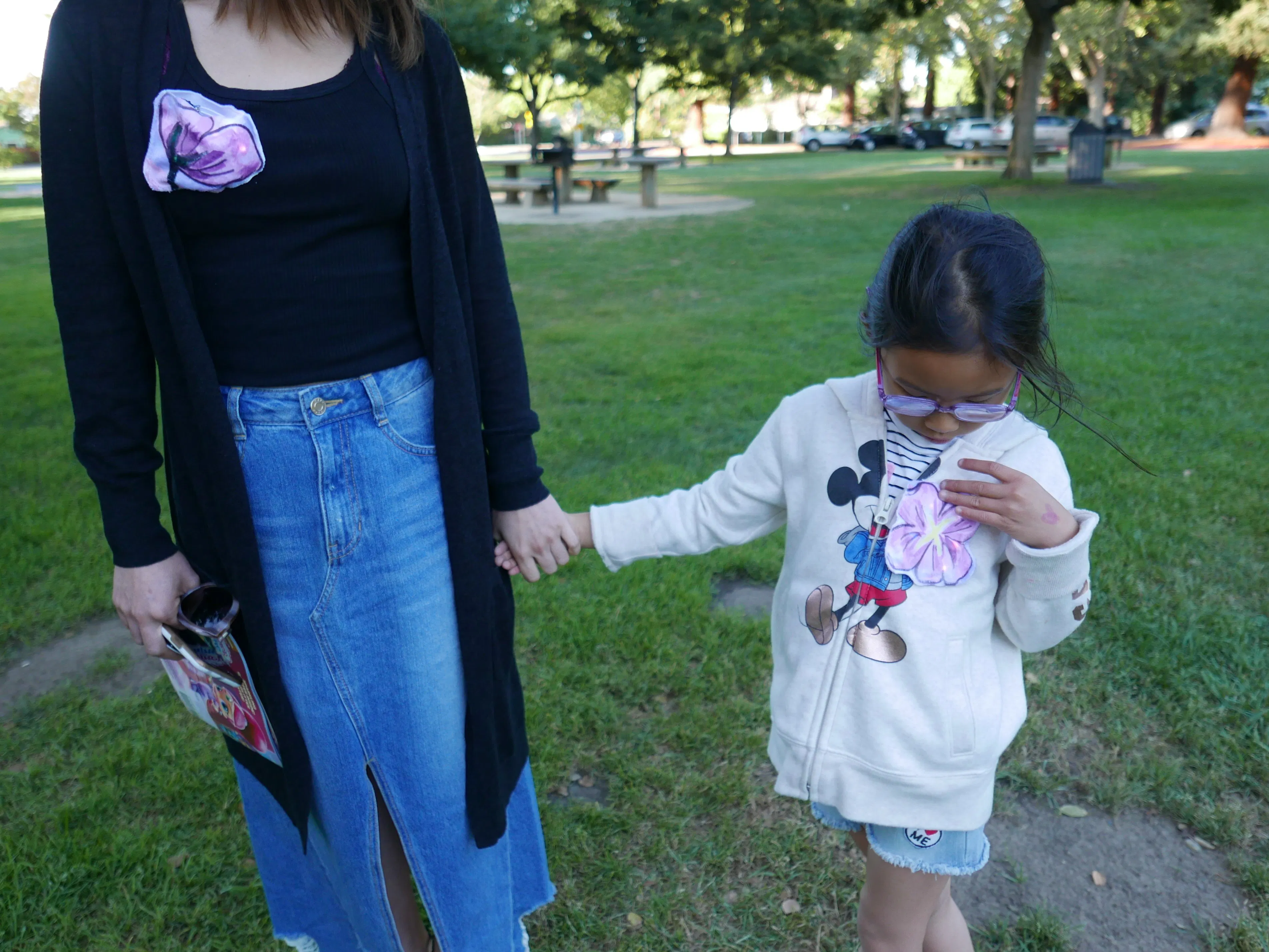## Things used in this project

### Hardware components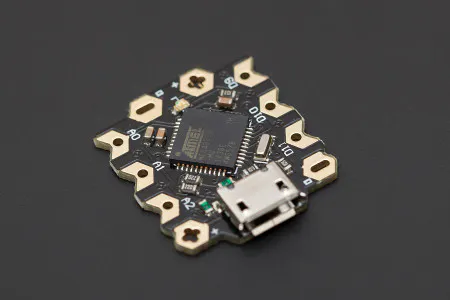DFRobot Beetle - The Smallest Arduino
×2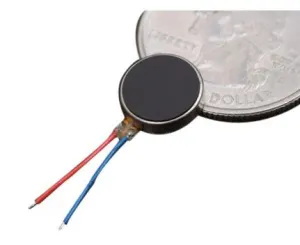Brown Dog Gadgets Solar Cockroach Vibrating Disc Motor
×1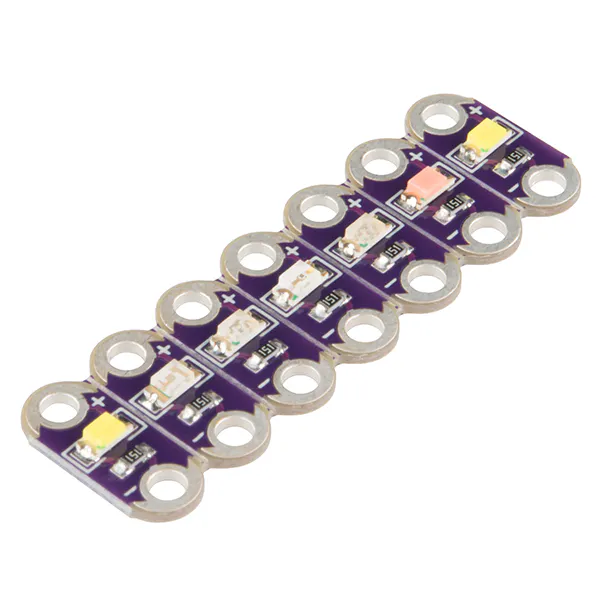SparkFun LilyPad Rainbow LED (6 Colors)
×2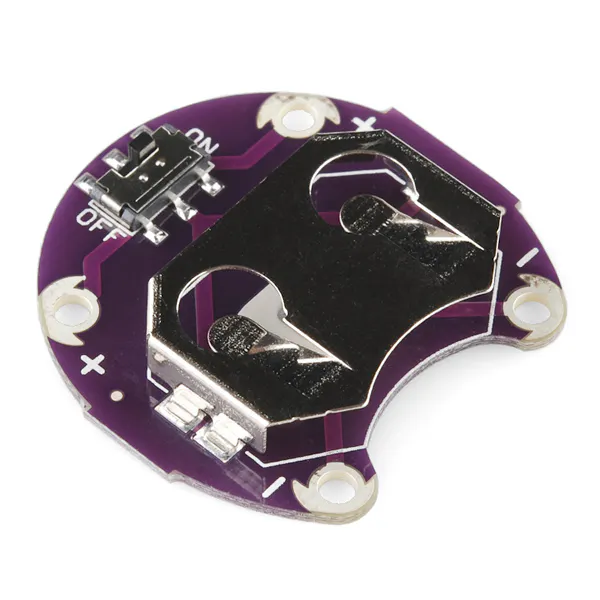SparkFun LilyPad Coin Cell Battery Holder - Switched - 20mm
×4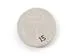Coin Cell Battery CR2032
×1

### Software apps and online servicesArduino IDE

### Hand tools and fabrication machinesSoldering iron (generic)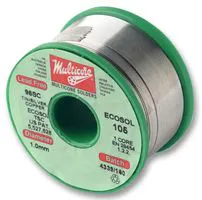## Schematics

### Central and Peripheral schematics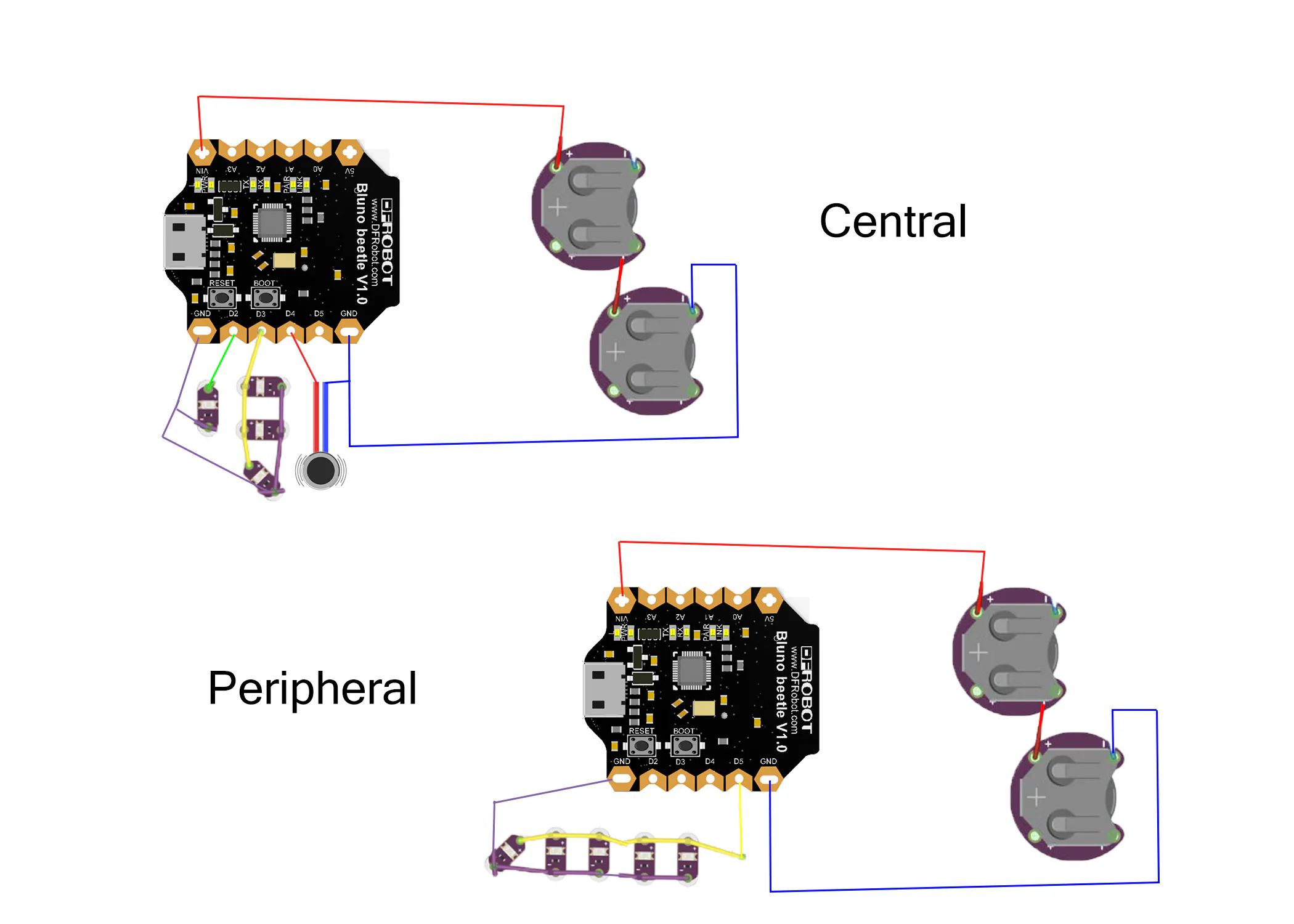## Code

### Central code

Arduino
```#include <SoftwareSerial.h>
//#include <LiquidCrystal_I2C.h>

//LiquidCrystal_I2C lcd(0x20,16,2);
SoftwareSerial mySerial(0, 1); // RX, TX

const int ledBLE1 = 2;
const int ledBLE2 = 3;
const int ledBLE3 = 4;
typedef enum {START, WAIT_AT, WAIT_RSSI, WAIT_EXIT, MODE_AT} state_type;

state_type state = MODE_AT;
char chr;
void mode_at();
void wait_exit();
void setup() {
mySerial.begin(115200);
Serial.begin(115200);      //Initiate the Serial comm
pinMode(ledBLE1, OUTPUT);
pinMode(ledBLE2, OUTPUT);
pinMode(ledBLE3, OUTPUT);
state = MODE_AT;
// lcd.init();                      // initialize the lcd
//lcd.backlight();

}

void loop() {
if(state==MODE_AT){
mySerial.println(1);
mode_at();
delay(3000);
}else if(state == START) {
delay(100);
start();
}else if(state == WAIT_AT) {
delay(100);
wait_at();}
delay(100);
}else if (state == WAIT_EXIT) {
wait_exit();
delay(3000);
}
}

void start() {
delay(10);
Serial.print("+++");
state = WAIT_AT;
}

void wait_at() {
while (Serial.available() > 0) {
if (chr == 'E') {
buffer = "";
}
if (chr == 'O'&&buffer!="ERR") {
buffer = "";
}
buffer += chr;
}
if (reading && buffer == "ERROR CMD\r\n") {
state = START;
}
if (reading && buffer == "Enter AT Mode\r\n") {
Serial.flush();
if (reading && buffer == "OK\r\n") {
Serial.flush();
}
}

while (Serial.available() > 0) {
if (chr == '-') {
buffer = "";
}
buffer += chr;
}
if (buffer.length() == 4) {
//lcd.clear();
//lcd.setCursor(0, 0);
// lcd.print("dBm=");
//mySerial.println(5);
digitalWrite(ledBLE1, HIGH);
digitalWrite(ledBLE2, LOW);
digitalWrite(ledBLE3, LOW);
}
digitalWrite(ledBLE1, LOW);
digitalWrite(ledBLE2, HIGH);
digitalWrite(ledBLE3, LOW);
}
else if (rssi >= "-070") {
digitalWrite(ledBLE1, LOW);
digitalWrite(ledBLE2, LOW);
digitalWrite(ledBLE3, HIGH);
}
state = WAIT_EXIT;
}
}
Serial.println("AT+EXIT");
Serial.flush();

}

void wait_exit(){
while (Serial.available() > 0) {
if (chr == 'O'&&buffer!="ERR") {
buffer = "";
}
if (chr == 'E') {
buffer = "";
}
buffer += chr;
}
mySerial.println(buffer);
if (reading && buffer == "ERROR CMD\r\n") {
if (buffer == "OK\r\n") {
state = START;
}
}
}

void mode_at() {
Serial.println("AT\n\n");
Serial.flush();
while (Serial.available() > 0){
if (chr == 'O'&&buffer!="ERR") {
buffer = "";}
if (chr == 'E'){
buffer = "";}
buffer += chr;}
mySerial.println(buffer);
if (reading && buffer == "ERROR CMD\r\n") {
state=MODE_AT;}
if(buffer == "OK\r\n") {
state = WAIT_AT;}
}
state = START;
}
```

### Peripheral code

Arduino
```#include <SoftwareSerial.h>
//#include <LiquidCrystal_I2C.h>

//LiquidCrystal_I2C lcd(0x20,16,2);
SoftwareSerial mySerial(0, 1); // RX, TX

const int ledBLE1 = 5;

typedef enum {START, WAIT_AT, WAIT_RSSI, WAIT_EXIT, MODE_AT} state_type;

state_type state = MODE_AT;
char chr;
void mode_at();
void wait_exit();
void setup() {
mySerial.begin(115200);
Serial.begin(115200);      //Initiate the Serial comm
pinMode(ledBLE1, OUTPUT);

state = MODE_AT;
// lcd.init();                      // initialize the lcd
//lcd.backlight();

}

void loop() {
if(state==MODE_AT){
mySerial.println(1);
mode_at();
delay(3000);
}else if(state == START) {
delay(100);
start();
}else if(state == WAIT_AT) {
delay(100);
wait_at();}
delay(100);
}else if (state == WAIT_EXIT) {
wait_exit();
delay(3000);
}
}

void start() {
delay(10);
Serial.print("+++");
state = WAIT_AT;
}

void wait_at() {
while (Serial.available() > 0) {
if (chr == 'E') {
buffer = "";
}
if (chr == 'O'&&buffer!="ERR") {
buffer = "";
}
buffer += chr;
}
if (reading && buffer == "ERROR CMD\r\n") {
state = START;
}
if (reading && buffer == "Enter AT Mode\r\n") {
Serial.flush();
if (reading && buffer == "OK\r\n") {
Serial.flush();
}
}

while (Serial.available() > 0) {
if (chr == '-') {
buffer = "";
}
buffer += chr;
}
if (buffer.length() == 4) {
//lcd.clear();
//lcd.setCursor(0, 0);
// lcd.print("dBm=");
//mySerial.println(5);
digitalWrite(ledBLE1, LOW);

}
digitalWrite(ledBLE1, HIGH);

}
else if (rssi >= "-070") {
digitalWrite(ledBLE1, LOW);

}
state = WAIT_EXIT;
}
}
Serial.println("AT+EXIT");
Serial.flush();

}

void wait_exit(){
while (Serial.available() > 0) {
if (chr == 'O'&&buffer!="ERR") {
buffer = "";
}
if (chr == 'E') {
buffer = "";
}
buffer += chr;
}
mySerial.println(buffer);
if (reading && buffer == "ERROR CMD\r\n") {
if (buffer == "OK\r\n") {
state = START;
}
}
}

void mode_at() {
Serial.println("AT\n\n");
Serial.flush();
while (Serial.available() > 0){
if (chr == 'O'&&buffer!="ERR") {
buffer = "";}
if (chr == 'E'){
buffer = "";}
buffer += chr;}
mySerial.println(buffer);
if (reading && buffer == "ERROR CMD\r\n") {
state=MODE_AT;}
if(buffer == "OK\r\n") {
state = WAIT_AT;}
}
state = START;
}
```

## Credits

### Kitty Yeung

9 projects • 110 followers
Physicist/Artist/Musician/Fashion Designer/Engineer www.kittyyeung.com
Thanks to Pearl.